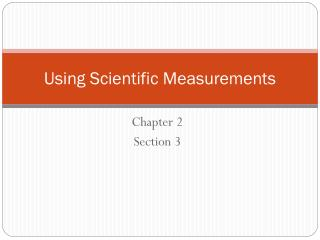DownloadDownload PresentationUsing Scientific Measurements

# Using Scientific Measurements

Download Presentation## Using Scientific Measurements

- - - - - - - - - - - - - - - - - - - - - - - - - - - E N D - - - - - - - - - - - - - - - - - - - - - - - - - - -
##### Presentation Transcript

1. Using Scientific Measurements Chapter 2 Section 3

2. Objectives • Distinguish between accuracy and precision • Determine the number of significant figures • Perform mathematical operations involving significant figures • Convert measurements into scientific notation • Distinguish between inversely and directly proportional relationships

3. Accuracy and Precision • (i) Accuracy: refers to how close an answer is to the “true” value • Generally, don’t know “true” value • Accuracy is related to systematic error • (ii) Precision: refers to how the results of a single measurement compares from one trial to the next • Reproducibility • Precision is related to random error Low accuracy, high precision Low accuracy, low precision High accuracy, low precision High accuracy, high precision

4. Significant Figures • Non-zero numbers are always significant. • For example, 352 g has 3 significant figures. • Zeros between non-zero numbers are always significant. • For example, 4023 mL has 4 significant figures. • Zeros before the first non-zero digit are not significant. • For example, 0.000206 L has 3 significant figures. • Zeros at the end of the number after a decimal place are significant. • For example, 2.200 g has 4 significant figures. • Zeros at the end of a number before a decimal place are ambiguous (e.g. 10,300 g).

5. Multiplying & Dividing Least # of sig figs in value Example: 4.870 x 3.21 15.6

6. Adding & Subtracting Least precise number--usually determined by Least # of decimal places Examples: 2.345 2500. + 0.1__+ 27.3 2.4 2527.

7. Rounding Rules If the first digit to be removed is 5 or greater, round UP, 4 or lower, round DOWN. Example: 2.453 rounded to 2 sig figs is 2.5 5.532 rounded to 3 sig figs is 5.53

8. Percent Error • Percent Error: • Measures the accuracy of an experiment • Can have + or – value

9. Example • Measured density from lab experiment is 1.40 g/mL. The correct density is 1.36 g/mL. • Find the percent error.

10. Density Used to characterize substances (a measure of “compactness”) and is an intensive property. Defined as mass divided by volume: Units: g/cm3. Sometimes this is written as g • cm–3. NOTE: cm3 = mL Frequently used as a conversion factor (mass to volume) Mass and volume are extensive properties, they are dependant on the amount of substance.

11. Bromine is one of two elements that is a liquid at room temperature (mercury is the other). The density of bromine at room temperature is 3.12 g/mL. What volume of bromine is required if a chemist needs 36 g for an experiment? Solution: 11.53 mL Sig fig 12 mL

12.   Volume from Mass and Density What volume is occupied by 461 g of mercury when it’s density is 13.6 g/ mL? Solution Here we can express the inverse of density as a ratio, 1.00 mL/13.6 g, and use it as a conversion factor. V= 461 g x ––––– = 3.9 mL 1 mL 13.6 g

13. A metal ball was found to have a mass of 0.085 kg and a volume of 3.1 mL. Calculate the density of the metal ball in units of g/mL.

14. The density of liquid mercury is 13.55 g/cm3. A mercury thermometer contains exactly 0.800 mL of liquid mercury. Calculate the mass of the liquid mercury contained in the thermometer.

15. A glass container weighs 48.462 g. A sample of 4.00 mL of antifreeze solution is added, and the container plus the antifreeze weigh 54.51 g. Calculate the density of the antifreeze solution.